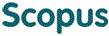### BROWSE

12 316

Cited 0 times in침수시 평균체온의 측정에 관한 연구

Other Titles
Estimation of mean body temperature during immersion in man
Authors
박성환
Issue Date
1982
Description
의학과/박사
Abstract
[한글] 평균체온(Τ^^B)은 심부온도(직장온도; T^^R)와 평균피부온도(Τ^^s) 및 이들 온도를 대표하는 체적비로부터 산출되는데 Burton(1935)이 human calorimetry를 통하여 측정한 바에 의하면 Τ^^B =0.65T^^R + 0.35Τ^^s 로 표시된다. 그러나 사람을 물속에 침수시킬때 이 심부온도체적비(core fraction; a)가 어떻게 되는지, 또 수온과 침수시간에 따라 어떻게 변하는지를 규명하고져 본 실험을 시행하였다. 평균체온을 구하는 이론적인 배경은 다음과 같다(Burton, 1935). Τ^^B = aT^^R + (1-a)Τ^^s △Τ^^B = (△H)/(B.W.×0.83) 단 △H = heat production-heat loss △Τ^^B : 평균체온의 변화 △H : Heat content change 본 실험에 참여한 피검자는 건강한 남자6명을 대상으로 하였고 실험방법에서 heat prod uction은 spirometer를 이용한 indirect calorimetry 법으로 측정하였고 heat loss는 단열재로 둘러싼 수조를 이용하여 direct calorimetry법으로 측정하였다. 이때, 수온은 15, 22, 및 25℃와 T^^cw(critical water temperature: 29∼31℃)로 하였다. 실험 결과를 요약하면 다음과 같다. 1. 직장온도는 15℃ 수온의 경우를 제외하고는 별 변동이 없었다. 2. 체열생산율 및 체열소실율은 수온이 낮을수록 높았다. 3. 평균체온은 수온이 낮을수록 심하게 감소하며 20분까지는 크게 감소하다가 그 이후 는 약간 서서히 감소하였다. 4. 침수시 심부온도체적비는 모든 수온에서 Burton(1936)이 발표한 0.65 보다 높았으며 수온이 낮을수록 더 높게 나타났으며 대체로 30분후 평형에 도달하였다. 5. 평형에 도달한 후 수온에 따른 심부온도체적비를 plot해 볼때 Y=-0.0067x+0.847(r=0.998, n=4)의 직선관계로 표시할 수 있다. 이상의 실험결과로서 이제까지의 평균체온의 산출에 있어서 인체가 공기에 노출되었을 때 core fraction의 값 0.65를 이용했던 Burton (1935)의 공식은 수중에 침수되었을 때에는 적용되지 않으며 수중에서의 평균체온산출에서도 이에 수정을 가하지 않으면 안될 것 으로 사료된다.
[영문] In many clinical and experimental situations the mean body temperature of a human is customary estimated using the following formula reported first by Burton(1935): Τ^^B = 0.65 T^^R +0.35Τ^^s Where, Τ^^B = mean body temperature T^^R = rectal temperature Τ^^s = mean skin temperature 0.65 and 0.35=fraction of body mass represented by the rectal and the mean skin temperature, respectively. Although this formula has been preyed to be adequate in the estimation of the mean body temperature of an indivisual exposed in air, its applicability when an indivisual is immersed in water has never been verified. It is well recognized that the human body cools faster in water than in air of the same temperature, mainly due to relatively high heat capacity and heat conductivity of water. It is therefore possible that the core fraction measured in water may not be identical with that in air. Therefore the present study was conducted to evaluate the changes in core fraction of human subjects immersed in water of various temperatures. In 6 healthy male subjects the fraction of core was estimated from the mean body temperature, using the following formula: Τ^^Bn = a^^n T^^Rn + (1-a^^n )T^^Sn = Τ^^Bn -1-△Τ^^Bn where, a^^n = core fraction at time n T^^Rn and Τ^^Sn = rectal and mean skin temperatures at time n Τ^^Bn and Τ^^Bn -1 = mean body temperature at time n and n-1 △Τ^^Bn = mean body temperature change between time n-1 and n Changes in mean body temperature(△Τ^^B) were estimated from the heat production and heat loss, using the following formula: Τ^^B = (△H)/(0.83×B.W) where, △H: change in body heat content over the specified time period 0.83: specific heat of human body in Kcal/℃/kg B.W.: body weight in kg The difference between heat production and heat loss baa taken as the change in body heat content over the same period: △H = Heat production-Heat loss The heat production was estimated from the oxygen consumption and the heat loss was determined by the direct calorimetric technique. The results are summarized as follows: 1. While immersion in water of 15℃ the rectal temperature of the subject declined continuously with time, but in water of 22℃, 25℃ and approximately 30℃(critical water temperature) it did not appreciably alter. 2. The heat production and the heat loss increased as water temperature lowered. 3. Regardless of water temperature the mean body temperature fell quickly during the initial 20 minutes period of immersion, then declined slowly thereafter. However, the degree of reduction was invesely proportioned to the water temperature. 4. At all temperatures the core fraction declined with immersion time during the first 30 minutes, after which it leveled off. The steady-state value of core fraction appeared to be inversely proportional to the water temperature (y=-0.0067κ+0.847, r=-0.998, n=4), and this value was consistantly abode 0.65 at all temperatures tested. These results indicate that the Burton's formula of mean body temperature is not directly applicable to the subject in water.
Full Text
https://ymlib.yonsei.ac.kr/catalog/search/book-detail/?cid=CAT000000007736
Files in This Item:
제한공개 원문입니다.
Appears in Collections:
1. College of Medicine (의과대학) > Others (기타) > 3. Dissertation
URI
https://ir.ymlib.yonsei.ac.kr/handle/22282913/115847
사서에게 알리기